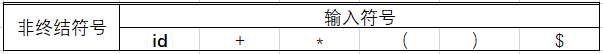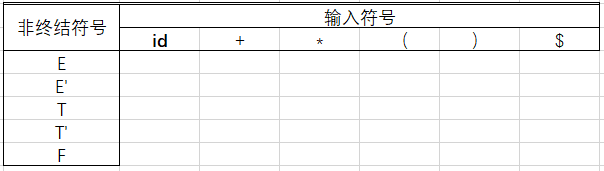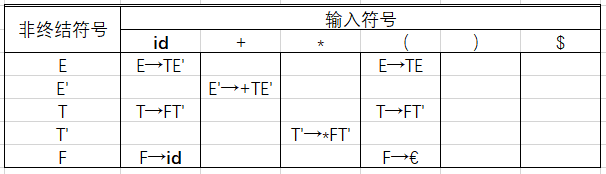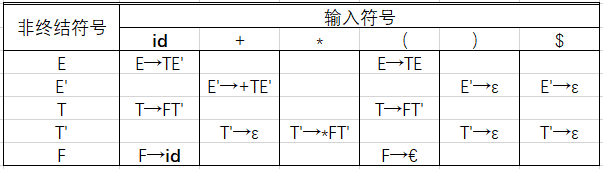• 语言为C++，使用了set，map容器，输入格式：S -> Aa | g | e，支持多‘|’ 符号，采用文件输入
• 通过课程设计进一步理解高级语言在计算机中的执行过程，加深对编译原理中重点算法和编译技术的理解，提高自己的编程能力，培养好的程序设计风格。同时通过某种可视化编程语言的应用，具备初步的Windows环境下的编程...编译原理
• ## FIRST集合基本构造

千次阅读 2020-05-16 23:05:31
FIRST集合 定义 令G是一个不含左递归的文法，对G的所有非终结符的每个候选a定义它的终结首符集FIRST(a)为： FIRST(α) = { a│α ⇒∗ a…, a∈VT } 若α⇒∗ε，则规定ε∈FIRST(α)。 FIRST(α)被定义为从...
FIRST集合
定义

令G是一个不含左递归的文法，对G的所有非终结符的每个候选a定义它的终结首符集FIRST(a)为：
FIRST(α) = { a│α ⇒∗ a…, a∈VT }
若α ⇒∗ ε ，则规定ε∈FIRST(α)。

FIRST(α)被定义为从α推导得到的句子的首符号的集合，α是任意的文法符号串。
n非终结符的FISRT集合中有空串与该非终结符属于NULLABLE集合等价。
FIRST集合构造
构造每个文法符号的FIRST集合

第一种说明：
对于文法G的每个文法符号X∈VT∪VN：
若 X ∈ VT，则 FIRST(X) = { X }若 X ∈ NULLABLE，则把 ε 加入FIRST(X)若 X ∈ VN，且 X → a…, a ∈ VT，则把a加入到FIRST(X)中若 X ∈ VN，且 X → Y…, Y ∈ VN，则把FIRST(Y) - {ε}加到FIRST(X)中若 X →Y1Y2 … Yi , 且 Y1, Y2, … ,Yi-1 ∈ nullable，则把 FIRST(Yi) - {ε}加到FIRST(X)中若 FIRST(Ym) ∈ NULLABLE(1<=m<=i)，则ε∈FIRST(X)

第二种说明：
对每一X∈VT∪VN，连续使用下面的规则，直至每个集合FIRST不再增大为止：
若X ∈ VT，则FIRST(X)＝{X}。若X ∈ VN，且有产生式X→a…，则把a加入到FIRST(X)中；若X→ε也是一条产生式，则把 ε 也加到FIRST(X)中。若X→Y…是一个产生式且Y ∈ VN，则把FIRST(Y)中的所有非 ε- 元素都加到FIRST(X)中；若X→Y1Y2…Yi-1Yi…Yk是一个产生式，Y1，…，Yi-1都是非终结符，对于任何j，1<=j<=i-1，FIRST(Yj)都含有 ε (即Y1…Yi-1 ⇒∗ε)， 则把FIRST(Yi)中的所有非 ε-元素都加到FIRST(X)中；若所有的FIRST(Yj)均含有 ε，j＝1，2，…，k，则把 ε 加到FIRST(X)中。

如果上面两个构造方法看不懂也不要紧，我们大白话讲一下
第三种说明（这种比较好理解）：
对于 X -> ... 这条产生式而言，
若右边第一个符号是终结符或 ε  ，则直接将其加入 FIRST（X）若右边第一个符号是非终结符，则将其 FIRST 集的的非 ε 元素加入 FIRST（X）若右边第一个符号是非终结符而且紧随其后的是很多个非终结符，这个时候就要注意是否有 ε  。若第 i 个非终结符的 FIRST 集有 ε  ，则可将第 i+1 个非终结符去除 ε 的 FIRST 集加入 FIRST（X）。若所有的非终结符都能够推导出 ε ，则将  ε  也加入到 FIRST（X）

以上三种说明大致意思都是相同的

例题
文法G:
E → TE'
E' → +TE'│ε
T → FT'
T' → *FT'│ε
F → (E)│i
求每个非终结符号的FIRST集合
这是一道非常经典的例题，下面我们一起来看一下
因为FIRST集合是要不断重复进行的，我们可以多次循环构造它（以下均使用第三种说明）

第一次循环

//E的产生式紧跟的是两个非终结符，根据构造法第二条，FIRST(E)=FIRST(T),而FIRST(T)我们还没求出来，所以我们这项暂时为空
FIRST(E) = { }

//E'的产生式紧跟的是非终结符+，根据构造法第一条，将其加入到FIRST(E')中，产生式中还可以推导出ε，将ε也加入到FIRST(E')中
FIRST(E') = {+，ε}

//T的产生式紧跟的是FT'两个非终结符，根据构造法第二条，FIRST(T)=FIRST(F),而FIRST(T)我们还没求出来，所以我们这项暂时为空
FIRST(T) = { }

//T'的产生式紧跟的是非终结符*，根据构造法第一条，将其加入到FIRST(T')中，产生式中还可以推导出ε，将ε也加入到FIRST(T')中
FIRST(T') = {*，ε}

//F的产生式紧跟的是非终结符(，根据构造法第一条，将其加入到FIRST(T')中，产生式中还可以推导出i，将i也加入到FIRST(F)中
FIRST(F) = {(, i}

//第一次循环之后，有FIRST集合发生改变，再次循环，直到所有FIRST集合不发生改变为止

第二次循环

FIRST(E) = { }            //没有得到FIRST(T)，依旧不能得到FIRST(E)，所以为空

FIRST(E') = {+，ε}        //不变

FIRST(T) = {(, i}
//T的产生式紧跟的是非终结符F，根据构造法第二条，将FIRST(F)中的元素加入到FIRST(T)中

FIRST(T') = {*，ε}        //不变

FIRST(T) = {(, i}        //不变

//第二次循环之后，FIRST集合发生变化，再次循环

第三次循环

FIRST(E) = {(, i}          //根据构造法第二条，将将FIRST(T)中的元素加入到FIRST(E)中

FIRST(E') = {+，ε}         //不变

FIRST(T) = {(, i}        //不变

FIRST(T') = {*，ε}        //不变

FIRST(T) = {(, i}        //不变

//第三次循环之后，FIRST集合发生改变，接着循环

第四次循环

FIRST(E) = {(, i}

FIRST(E') = {+，ε}

FIRST(T) = {(, i}

FIRST(T') = {*，ε}

FIRST(T) = {(, i}

所有FIRST集合都不改变，完成FIRST集合的构造
构造任意符号串的FIRST集合

对文法G的任何符号串α=X1X2…Xn构造集合FIRST(α)

FIRST(α)={ a│α⇒∗ a…, a∈VT }
α = X， X∈VT∪VN
α = X1X2…Xn， X∈VT∪VN

1. 置FIRST(α)＝FIRST(X1) \ {ε}；
2. 若对任何1<=j<=i-1，ε∈FIRST(Xj)，则把FIRST(Xi) \ {ε}加至FIRST(α)中；特别是，若所有的FIRST(Xj)均含有ε，1<=j<=n，则把ε 也加至FIRST(α)中。显然，若α＝ε则FIRST(α)＝{ ε }。

如果能理解上面对文法符号的构造，对任意符号串的构造就很好理解了

如有错误，欢迎指正！
展开全文语法分析
• 里面是编译原理课上所讲的求first集合的源代码，使用C++编写的
• 求出所有文法符号的FIRST集合算法 1． 若X ∈ Vt，则FIRST（X）={X}， 2． 若X ∈Vn，并且有产生式 X→a ，则a ∈ FIRST(X) 3． 若X ∈Vn, 且有产生式X→ε，则ε∈FIRST(X). 4． 若X ∈Vn, 且有产生式X→Y1Y2….Yn...
• ## FIRST集合、FOLLOW集合以及LL(1)文法

万次阅读 多人点赞 2019-04-19 13:49:41
FIRST集合 定义 可从α推导得到的串的首符号的集合，其中α是任意的文法符号串。 规则 计算文法符号 X 的 FIRST(X)，不断运用以下规则直到没有新终结符号或 ε可以被加入为止 ： （1）如果 X 是一个终结符号，那么 ...
FIRST集合
定义
可从α推导得到的串的首符号的集合，其中α是任意的文法符号串。
规则
计算文法符号 X 的 FIRST(X)，不断运用以下规则直到没有新终结符号或 ε可以被加入为止 ：
（1）如果 X 是一个终结符号，那么 FIRST(X) = X。
（2）如果 X 是一个非终结符号，且 X ->Y1 Y2 … Yk是一个产生式，其中 k≥1，那么如果对于某个i，a在 FIRST(Y1）、FIRST(Y2）… FIRST(Yi-1）中，就把a加入到 FIRST(X) 中。
（3）如果 X ->ε是一个产生式，那么将ε加入到 FIRST(X)中。

以上是书上的官方规则，不仅读起来很拗口，理解也很累。
下面看一下精简版的规则（从别人 @樱草书  那里看来的，感觉很棒，这里引用一下）： （1）如果X是终结符，则FIRST(X) = { X } 。 （2）如果X是非终结符，且有产生式形如X → a…，则FIRST( X ) = { a }。 （3） 如果X是非终结符，且有产生式形如X → ABCdEF…（A、B、C均属于非终结符且包含 ε，d为终结符），需要把FIRST( A )、FIRST( B )、FIRST( C )、FIRST( d )加入到 FIRST( X ) 中。 （4）如果X经过一步或多步推导出空字符ε，将ε加入FIRST( X )。
实践
记得，曾经有人说过：

只读，就会白给

下面以这个文法为例讲解一波，会用精简版规则，更容易理解一些：
E -> T E'
E' -> + T E' | ε
T -> F T'
T' -> * F T' | ε
F -> ( E ) | id

FIRST(E) = FIRST(T) 根据规则3，很容易理解，这里要注意的由于T不含ε，所以遍历到T就停止了，E’不会加入进来FIRST(E’) = FIRST(+) ∪ FIRST(ε)= { +, ε } 根据规则2和4，,很好理解FIRST(T) = FIRST(F) 根据规则3，和第一条推导过程一样FIRST(T’) = FIRST() ∪ FIRST(ε)= { , ε } 根据规则2和4，和第二条推导一样FIRST(F) = FIRST( ( ) ∪ FIRST(id)= { ( , id } 根据规则2
结果：
FIRST(E) = FIRST(T) = FIRST(F) = { ( , id }
FIRST(E') = FIRST(+) ∪ FIRST(ε)= { + ,  ε }
FIRST(E') = FIRST(*) ∪ FIRST(ε)= { * ,  ε }

FOLLOW集合
定义
对于非终结符号A，FOLLOW（A） 被定义为可能在某些句型中紧跟在A右边的终结符号集合。
规则
计算文法符号 X 的 FOLLOW(X) ，不断运用以下规则直到没有新终结符号可以被加入任意FOLLOW集合为止 ：
（1）将$加入到FOLLOW(X)中，其中S是开始符号，而$是输出右端的结束标记。
（2）如果存在一个产生式S->αXβ，那么将集合FIRST(β)中除ε外的所有元素加入到FOLLOW(X)当中。
（3）如果存在一个产生式 S->αX , 或者S->αXβ且FIRST(β)中包含ε , 那么将集合FOLLOW(S)中的所有元素加入到集合FOLLOW(X)中。
实践
还是用之前的例子来做
E -> T E'
E' -> + T E' | ε
T -> F T'
T' -> * F T' | ε
F -> ( E ) | id

FOLLOW(E) ，根据规则1，首先把$加入进来，根据规则2，可以得出 FOLLOW(E) = { ) ,$ }FOLLOW(E’) = FOLLOW(E) = { ) , $} 根据规则3FOLLOW(T) = FIRST(E’) ∪ FOLLOW(E) = { + , ) ,$ } 根据规则2FOLLOW(T’) = FOLLOW(T) = { + , ) , $} 根据规则3FOLLOW(F) = FOLLOW(T) ∪ FIRST(T’) = { * , + , ) ,$ } 根据规则2和3
结果：
FOLLOW(E) = FOLLOW(E') = { ) , $} FOLLOW(T) = FOLLOW(T') = { + , ) ,$ }
FOLLOW(F) = { * , + , ) , $}  LL(1)文法 解释 LL(1) 中第一个“L”表示从左向右扫描输入，第二个“L”表示产生最左推导，而“1”表示在每一步中只需要向前看一个输入符号来决定语法分析动作。 定义 对于文法LL(1)文法G，当且仅当G的任意两个不同产生式 A -> α | β （1）不存在终结符号a使得α和β都能推导出以a开头的串。 （2）α和β中最多只有一个可以推导出空串。 （3）如果 β=》ε ，那么α不能推导出任何以FOLLOW(A)中某个终结符号开头的串。 可能很多人看的云里雾里，解释一下： （1）和（2）意思是α和β的FIRST集合相交。（3）是指如果FIRST(α)中有 ε，那么FIRST(β)和FOLLOW(A)是不相交的集合，反之一样。 预测分析表的构建 方法： 对于文法G的每个产生式 A->α ，进行如下处理 （1）对于FIRST(α)中每个终结符号a，将 A->α 加入到 M[A,a] 中。 （2）如果 ε在FIRST(α)中，那么对于FOLLOW(A)中每个终结符号b，将 A->α 加入到 M[A,b] 中。如果 ε在FIRST(α)，且$在FOLLOW(A)中，也将 A->α 加入到 M[A,$] 中。 还是以之前的例子示例 E -> T E' E' -> + T E' | ε T -> F T' T' -> * F T' | ε F -> ( E ) | id  1.先求FIRST和FOLLOW集合： FIRST(E) = FIRST(T) = FIRST(F) = { ( , id } FIRST(E') = FIRST(+) ∪ FIRST(ε)= { + , ε } FIRST(T') = FIRST(*) ∪ FIRST(ε)= { * , ε } FOLLOW(E) = FOLLOW(E') = { ) ,$ }
FOLLOW(T) = FOLLOW(T') = { + , ) , $} FOLLOW(F) = { * , + , ) ,$ }

2.然后构建一个这样的表3.然后依次填入非终结符号4.按照规则1填写其余内容5.按照规则2填写内容至此整个构建全部完成
展开全文编译原理 FOLLOW集合 LL(1)文法 预测分析表
• ## 【编译原理】FIRST集合和FOLLOW集合

千次阅读 多人点赞 2020-06-07 05:03:28
FIRST集合 定义：可从α推导得到的串的首符号的集合，其中α是任意的文法符号串。 规则：计算文法符号 X 的 FIRST(X)，不断运用以下规则直到没有新终结符号或 ε可以被加入为止 ： （1）如果 X 是一个终结符号...
FIRST集合

定义：可从α推导得到的串的首符号的集合，其中α是任意的文法符号串。

规则：计算文法符号 X 的 FIRST(X)，不断运用以下规则直到没有新终结符号或 ε可以被加入为止 ：
（1）如果 X 是一个终结符号，那么 FIRST(X) = X。
（2）如果 X 是一个非终结符号，且 X ->Y1 Y2 … Yk是一个产生式，其中 k≥1，那么如果对于某个i，a在 FIRST(Y1）、FIRST(Y2）… FIRST(Yi-1）中，就把a加入到 FIRST(X) 中。
（3）如果 X ->ε是一个产生式，那么将ε加入到 FIRST(X)中。

上述为官方给出的计算FIRST集合的规则，不太好理解，接下来我将综合其他笔者总结的规则给出我理解的FIRST集合的规则。

（1）如果X是终结符，则FIRST(X) = { X } 。 （2）如果X是非终结符，且有产生式形如X → a…，则FIRST( X ) = { a }。 （3） 如果X是非终结符，且有产生式形如X → ABCdEF…（A、B、C均属于非终结符且包含 ε，d为终结符），需要把FIRST( A )、FIRST( B )、FIRST( C )、FIRST( d )加入到 FIRST( X ) 中。 （4）如果X经过一步或多步推导出空字符ε，将ε加入FIRST( X )。

下面给出文法示例并做讲解:

E -> T E' E' -> + T E' | ε T -> F T' T' -> * F T' | ε F -> ( E ) | id

FIRST(E) = FIRST(T) 根据规则3，很容易理解，这里要注意的由于T不含ε，所以遍历到T就停止了，E’不会加入进来 FIRST(E’) = FIRST(+) ∪ FIRST(ε)= { +, ε } 根据规则2和4，,很好理解 FIRST(T) = FIRST(F) 根据规则3，和第一条推导过程一样 FIRST(T’) = FIRST() ∪ FIRST(ε)= { , ε } 根据规则2和4，和第二条推导一样 FIRST(F) = FIRST( ( ) ∪ FIRST(id)= { ( , id } 根据规则2
结果：
FIRST(E) = FIRST(T) = FIRST(F) = { ( , id }
FIRST(E') = FIRST(+) ∪ FIRST(ε)= { + , ε }
FIRST(T') = FIRST(*) ∪ FIRST(ε)= { * , ε }

FOLLOW集合

定义：对于非终结符号X，FOLLOW（X） 被定义为可能在某些句型中紧跟在A右边的终结符号集合。

规则：计算文法符号 X 的 FOLLOW(X) ，不断运用以下规则直到没有新终结符号可以被加入任意FOLLOW集合为止 ：
（1）将#加入到FOLLOW(X)中，其中S是开始符号，而#是输出右端的结束标记。
（2）如果存在一个产生式S->αXβ，那么将集合FIRST(β)中除ε外的所有元素加入到FOLLOW(X)当中。
（3）如果存在一个产生式 S->αX , 或者S->αXβ且FIRST(β)中包含ε , 那么将集合FOLLOW(S)中的所有元素加入到集合FOLLOW(X)中。

理解：FOLLOW集合对于非终结符而言，是非终结符的全部后跟符号的集合，
如果后跟终结符则加入，
如果后跟非终结符，则加入该非终结符的不含空符号串的FIRST集，
特别地，文法的识别符的FOLLOW集需要额外的加入‘#’。

下面给出简化后的规则：

首先：如果要找L的Follow，要从式子的右边找到L，然后来找L的Follow。
其次：将‘#’加入到 开始符 的 Follow中  ①
接着：如果L的右边是终结符，那么这个终结符加入L的Follow  ②
如果L的右边是非终结符， 那么把这个非终结符的First集合除去 ε后剩下的元素加到L的Follow中，同时将 '->' 符号左边的Follow加入L的Follow  ③
特别的，如果L处在末尾，那么，将 '->' 符号左边的Follow加入L的Follow  ④

练习：还是用之前的例子来做

1 |  E -> T E' 2 |  E' -> + T E' | ε 3 |  T -> F T' 4 |  T' -> * F T' | ε 5 |  F -> ( E ) | id

FOLLOW(E) ，运用 ① ，E是开始符，所以首先将 # 加入到 FOLLOW(E)中 ；接下来在上述文法的右边找到  E ，发现E在第5行文法的右边，E 后面是终结符 ) ，所以将 ）加入到 FOLLOW(E)中 ；此处运用的是规则②，综上即 FOLLOW(E) = { ) , # }
FOLLOW(E’) ，先在上述文法的右边找到  E' ，发现E' 分别在第1行（在自己行不算），此时可以运用规则④，即将FOLLOW(E) 加入到FOLLOW(E')中，综上即 FOLLOW(E')= FOLLOW(E) = { ) , # }
FOLLOW(T)，先在上述文法的右边找到 T ，发现T在第一行，此时运用规则 ③，将E'的FIRST集合中除  ε外其他元素加入到FOLLOW(T)中，同时将FOLLOW(E) 加入到FOLLOW(T)中，综上即FOLLOW(T)= { + , ) , # }
FOLLOW(T’) ，先在上述文法的右边找到 T ' ，发现T'在第三行，此时可以运用规则④，即将FOLLOW(T) 加入到FOLLOW(T')中，综上即 FOLLOW(T')= FOLLOW(T) = { + , ) , # }
FOLLOW(F),先在上述文法的右边找到 F ，发现F在第三行和第四行，此时运用规则 ③，对于第3行，将T'的FIRST集合中除  ε外其他元素加入到FOLLOW(F)中，同时将FOLLOW(T) 加入到FOLLOW(F)中，此时FOLLOW(F)= { * , + , ) , # } ；对于第4行,将将T'的FIRST集合中除  ε外其他元素加入到FOLLOW(F)中，同时将FOLLOW(T') 加入到FOLLOW(F)中，而 FOLLOW(T')= FOLLOW(T)，所以FOLLOW(F)依旧= { * , + , ) , # } ，综上所述，FOLLOW(F)= { * , + , ) , # } 。
结果：
FOLLOW(E) = FOLLOW(E') = { ) , # }
FOLLOW(T) = FOLLOW(T') = { + , ) , # }
FOLLOW(F) = { * , + , ) ,# }

FIRST集合和FOLLOW集合在编译原理语法分析中是我觉得比较难理解的，以上是我看过很多csdn上面其他文章再加上我的理解总结出来的，尤其是FOLLOW集合，是我看了另一篇写的好的文章加上我的理解总结出来的，希望对你们有帮助。
展开全文• ## FIRST集合求法

千次阅读 2020-06-07 13:50:48
FIRST集求法 FIRST集的定义 ...First（A）集的作用是标示在替换非终结符A的时候，替换后的文法的首字母集合，语法分析程序根据这个来判断给定的语言是否是合法的，是符合规则的。 FIRST 集求法 ...
FIRST集合求法
FIRST集的定义 定义：令X 为一个文法符号（一个终结符或非终结符）或ε，则集合First (X) 由从X可能推导出的所有终结符号串的开头终结符组成，此外可能还有ε，它的定义如下：

（1）若X 是终结符或ε，则First (X) = {X}。 （2）若X 是非终结符，则对于每个产生式X→X1X2. . . Xn ，First (X)都包含了First(X1) - {ε}。 若对于某个i < n ，所有的集合First (X1), . . . , First (Xi) 都包括了。则First (X) 也包括了First (X i + 1 ) -{ε}。若所有集合First (X1), . . . , First (Xn)包括了ε，则First (X)也包括ε。

现在为任意串a = X1 X2 . . . Xn （终结符和非终结符的串）定义First (a)，如下所示：First (a)包括First (X1) 对于每个i = 2, . . . , n ，如果对于所有的k = 1, . . . ,i -1 ，First (Xk) 包括了ε，则First (a)就包括了First (Xi)。最后，如果对于所有的i =1, . . . , n ，First (Xi) 都包括了ε，则First (a)也包括了ε。

FIRST集的作用 First（A）集的作用是标示在替换非终结符A的时候，替换后的文法的首字母集合，语法分析程序根据这个来判断给定的语言是否是合法的，是符合规则的。FIRST 集求法 利用定义计算，具体步骤如下: (1) 若X ∈ Vt，则FIRST(X) = {X}。(即定义1) (2）若X ∈ Vn，且有产生式X → a……， a ∈ Vt，则a ∈ FIRST(X) (非终结符，将首个终结符加入First集) (3）若X ∈ VN，X → ε，则 ε ∈ FIRST(X) (直接推导) (4）若X→Y1,Y2,……,Yn ∈ Vn，而有产生式X → Y1,Y2,……,Yn。当Y1,Y2,……,Y(i-1) 直接推出ε时，则FIRST(Y1) - ε, FIRST(Y2) - ε, …… , FIRST(Y(i-1) - ε) ,FIRST(Yi) 都包含在FIRST(X)中(无ε) (5）当(4）中所有Yi 都推出 ε时，则最后的FIRST(X) = FIRST(Y1) ∪ FIRST(Y2) ∪ …… ∪ FIRST(Yn) ∪ {ε} 反复运用(2）-(5）步骤计算，直到每个符号的FIRST集合不再增大为止

例如:文法： S→ABc A→a|ε B→b|ε 解: FIRST（A）=｛a，ε｝ FIRST（B）={b,ε} FIRST(S）=｛a，b，c｝

技巧： First一般从下往上找。 如果要找A的First，我们要找A的定义式，即A在左边的式子，看着他的右边来找。  例题 文法G[A] A→BCc|gDB B→bCDE|ε C→DaB|ca D→dD|ε E→gAf|c

FIRST(A)=FIRST(BCc)∪FISRT(gDB)

=(FIRST(B)-{ε})∪FIRST(Cc)∪{g} ={b,d,a,c,g}

FIRST(B)=FIRST(bCDE)∪FIRST(ε)

={b,ε}

FIRST( C)=FIRST(DaB)∪FIRST(ca)

=FIRST(dDaB)∪FIRST(aB)∪{c} ={d,a,c}

FIRST(D)=FIRST(dD)∪FIRST(ε) ={d,ε} FIRST(E)=FIRST(gAf)∪FIRST( c )={g,c}

展开全文算法
• 里面包含LL1文法的构造和First和follow集合的求解，有C语言写的，有用C#写的，有用VB写的~
• FIRST集合和FOLLOW集合的计算实例演示 自顶向下分析中一个重要步骤就是求解文法中各个终结符的FIRST集合和FOLLOW集合。下面我们通过一个具体的例子来练习FIRST和FOLLOW的计算。 不妨设一文法如下： E->TE’ E’-...
• FIRST集合 定义 可从α推导得到的串的首符号的集合，其中α是任意的文法符号串。 规则 计算文法符号 X 的 FIRST(X)，不断运用以下规则直到没有新终结符号或 ε可以被加入为止 ： （1）如果 X 是一个终结符号，...编译原理
• FIRST集合的求解规则 计算各个文法符号X的FIRST(X)时，不断应用下列规则，直到再没有新的终结符号或者ε可以被加入到任何FIRST集合中为止。 如果X是一个终结符号，那么FIRST(X) = X。 如果X是一个非终结符号...编译原理
• 看了好多求FIRST集和FOLLOW集的方法，这里是自己总结的，也是认为最实用的办法 FIRST集 求谁的FIRST集，要找谁在左侧的产生式，拿书上的例子来说（编译原理，陈火旺，第三版） E->TE' E'->+TE'|@ T->FT' T'...经验分享
• 输入产生式最后#结束 程序会输出各个非终结符的first集合
• 如果 X 是非终结符，则First集合一直传送下去，直到遇到终结符，第一个状态加入到First集合中。 FOLLOW 集合求法 对于一个非终结符 A： 如果A是起始符号，Follow(A) = \$. 如果有 B——> a A b，Follow(A) = ...
• 对于First集合，follow集合的构造方法，我引用的是国防科技大学王挺老师的方法，我觉得这个讲的比较详细易懂； 下面用一道例题进行应用； 求 E->T|E+T T->F|T*F F->i |(E)的所有产生式的Follow集合，所有...
• LL(1)文法判别之First集合、Follow集合、Select集合求法说明：所有大写字母代表非终结符，小写字母代表终结符，省略号代表未知数目（可能为0）的不确定类型的文法符号。First集合First集合顾名思义就是求一个文法...
• * 获取非终结符号的产生式的first集合X->Y1Y2Y3Y4Y5……这样的, * @param str X * @return */ public List<String> getFirstItem(Production production) { List<String> list = new ArrayList();// 获取包含...编译原理 java
• C++写的关于上下文无关文法LL1的First集合的一种实现，vs2010开发环境中诞生的
• 关于编译原理试验 中上下文无关文法求first集合以及follow集合LL（1）文法判断
• 已知文法 G(E)  E →T|E＋T T→F|T *F F →(E)|id 求所有非终结符的first集合和follow集合正则表达式
• FIRST集合、FOLLOW集合、SELECT集合以及预测分析表地构造 FIRST集合的简单理解就是推导出的字符串的开头终结符的集合。 FOLLOW集合简单的理解就对于非终结符后面接的第一个终结符。 给定一个由终结符和非终结...
• FIRST集产生式，是第一次写，还有很多不完善的地方，但是我学习到了很多，希望大家想学编译原理的可以看看我写的这个代码。matlab
• 为什么需要求FIRST集合：因为一个产生式存在多个候选式，选择哪一个候选式是不确定的，所以这就产生了回溯。回溯需要消耗大量的计算、存储空间，所以我们需要消除回溯。而消除回溯的其中一种方法叫作“预测”，即...
• 题目目的：熟练掌握自上而下的...编写First函数，实现其求解过程。 提示： 1， 非终结符为大写字母；或后面带'的大写字母 2， 终结符为 小写字母和符号（+、*）(#代表空串) 3， 推导符号为-> 4， 用end结束文法。 5，编译原理 c++
• 目的：熟练掌握自上而下的语法分析方法，并能用程序实现。...编写First函数，实现其求解过程。 提示： 非终结符为 大写字母；或 后面带’的大写字母 终结符为 小写字母和符号（+、*） 推导符号为-> 用...编译原理
• 技术还不是很成熟，希望大家不要嫌弃！程序是由java编写的，是看了以为前辈的程序有感而写的，也是为了交作业被逼的，呵呵！
•  (2)构造相应的FIRST集合和FOLLOW集合。  解析：首先，第一个式子，消除左递归。S->aFS’|+aFS'。S'->+aFS'|ε （此处的ε和e一样的 不同的书上印刷的不同）  然后，第二个式子，消除左因子。F->*aF...
• FIRSTFIRSTFIRST集合求法 对于形如X→a…X \to a \dotsX→a…，则将aaa添加进FIRST(X)FIRST(X)FIRST(X) 中 对于形如X→εX \to \varepsilonX→ε,则将ε\varepsilonε添加进FIRST(X)FIRST(X)FIRST(X) 中 对于形如X......